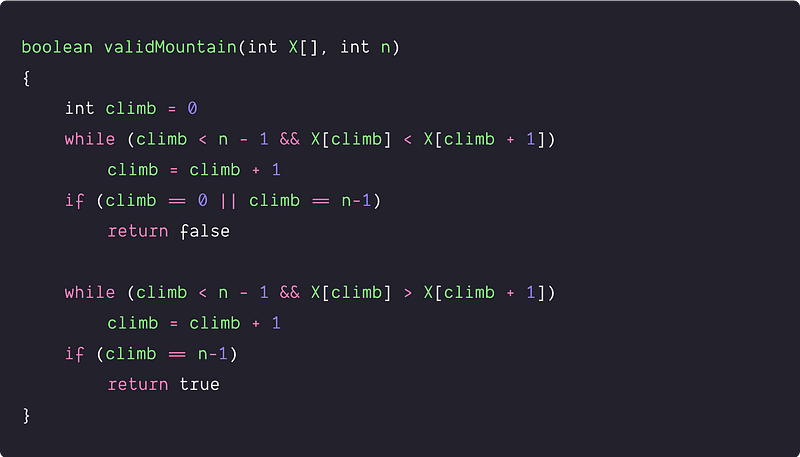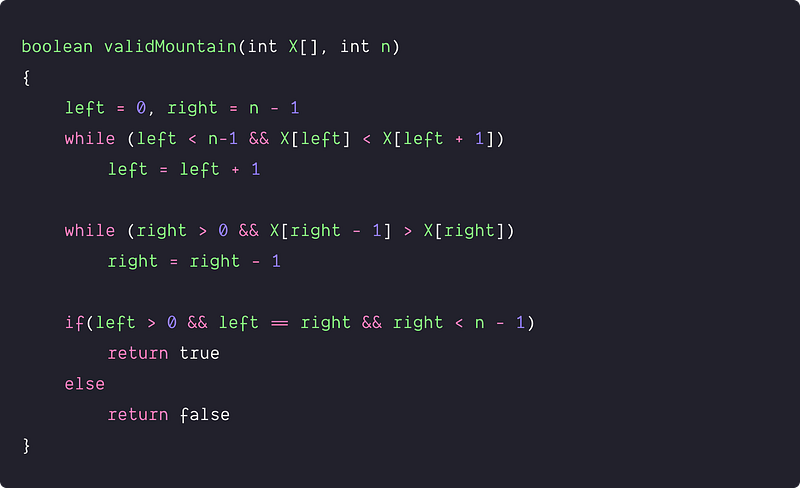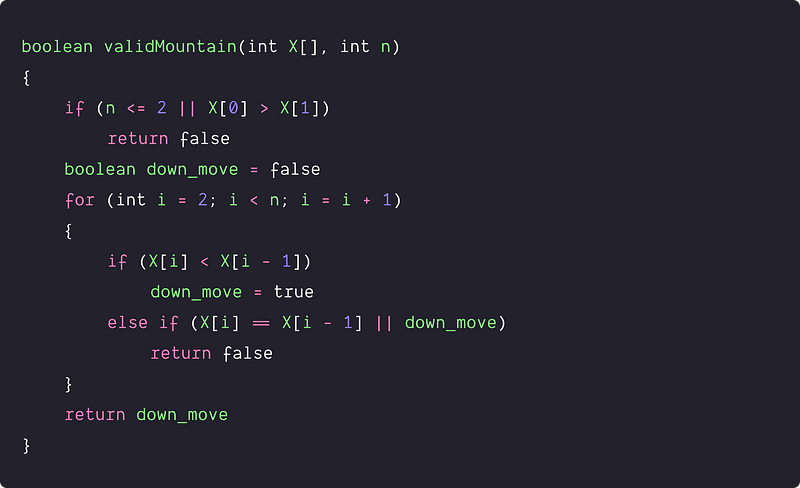# Valid Mountain Array

Key takeaway

• An excellent problem to learn problem-solving using a single scan
• Solution ideas are intuitive and easy to visualize
• A good interview problem for beginners to start

### Let’s understand the problem

Given an array X[] of n integers, write a program to check the given array is a valid mountain array or not.

• The array X[] is a mountain array if and only if n >= 3 and there exists some i (0 < i < n -1) such that: X < X <...X[i-1] < X[i] and X[i] > X[i+1] > ...> X[n-1].
• In other words, we call the array mountain array when the array is strictly increasing and then strictly decreasing.

Examples

Input: X[] = [5, 2, 1, 4], Output: false

Input: X[] = [5, 8, 8], Output: false

Input: X[] = [1, 2, 4, 2], Output: true

Important note: Before moving to the solutions, we recommend learners solve this problem. If solved, then well done! We would like to hear your ideas in the comment. Otherwise no problem, this is an opportunity to learn a new pattern in problem-solving!

### Discussed solution approaches

Solution 1: Traversing from left to right end

Solution 2: Traversing from both ends

### Solution 1: Traversing from left to right end

Solution Idea and Steps

If there is a mountain then we first move up from left to the peak and then move down from peak to the right. So the basic idea would be : scan the array from the left and check the strictly increasing and then decreasing order of elements.

• We start from the left end and initialise the variable climb to track the order of elements i.e. climb = 0.
• Now we check the strictly increasing order and reach the peak by running a loop. If X[climb] < X[climb + 1] and climb < n-1, then we are on the track of the increasing order and we move to the next element. We stop the loop if any one of the conditions becomes false.
• By end of the loop, if the peak is present at first or the last element i.e. climb = 0 or climb = n-1, then we return false. In other words, the peak can’t be the first or last element in the mountain.

``````int climb = 0
while (climb < n - 1 && X[climb] < X[climb + 1])
climb = climb + 1
if (climb == 0 || climb == n-1)
return false
``````
• If the peak is present at some middle element then similarly, we run a loop from that position to check the strictly decreasing order or elements. If we reach the end, the array is a valid mountain, otherwise, it’s not.

``````while (climb < n - 1 && X[climb] > X[climb + 1])
climb = climb + 1
if (climb == n-1)
return true
``````

Solution PseudocodeTime and space complexity analysis

In the worst case, we are performing a single scan of the array i.e we are accessing each element of the array only once. Time complexity = O(n). Space complexity = O(1) i.e no extra space is used.

### Solution 2: Traversing from both ends

Solution Idea and Steps

Here is another analogy of the solution. Suppose two people are climbing from the left and right end separately. If there is a valid mountain, then their first peak point will be the same. In other words, both will meet at the only peak point in the mountain array. Otherwise, if there is no valid mountain, then their first peak point would be different. (Think!)

• Initialise the variable left and right i.e. left = 0, right = n-1
• Using the loop, climb from the left end and reach the peak.

``````while (left < n-1 && X[left] < X[left + 1])
left = left + 1
``````
• Using the loop, climb the right end and reach the peak.

``````while (right > 0 && X[right - 1] > X[right])
right = right - 1
``````
• If (left > 0 && left == right && right < n — 1) then both are at the same peak of the mountain, return true. Otherwise return false.

Solution PseudocodeTime and space complexity analysis

In the worst case, we are traversing each element of the array only once. Time complexity = O(n), Space complexity = O(1) i.e no extra space is used.

Important note: We recommend learners transform the above pseudocodes into a favorite programming language (C, C++, Java, Python, etc.) and verify all the test cases. Enjoy programming!

### Critical ideas to think!

• Can we find another method to solve the problem by walking from left to right?
• are our solutions working for all test cases? What will happen in the case of repeated values?
• Can we solve this problem by counting the peak and valley in the given array? In the mountain array, Peak count = 1, Valley count = 0
• What would be the best, worst and average case analysis of 2nd approach?
• Here is a code snippet where we are tracking the down movement using a boolean variable down_move. Is this solution correct?### Comparisons of time and space complexities

• Traversing from left to right: Time Complexity = O(n), Space Complexity = O(1)
• Traversing from both end: Time Complexity = O(n), Space Complexity = O(1)

### Similar coding questions to practice

• Move zeroes to an end
• Number of buildings facing the sun
• Find the row with a maximum number of 1
• Find equilibrium index in an array
• Remove duplicates from sorted array
• Chocolate Distribution Problem
• Find minimum and maximum in an array
• Minimum Number of Removals to Make Mountain Array
• Find whether a subarray is in form of a mountain or not

Enjoy learning, Enjoy coding, Enjoy algorithms!

Get well-designed application and interview centirc content on ds-algorithms, machine learning, system design and oops. Content will be delivered weekly.